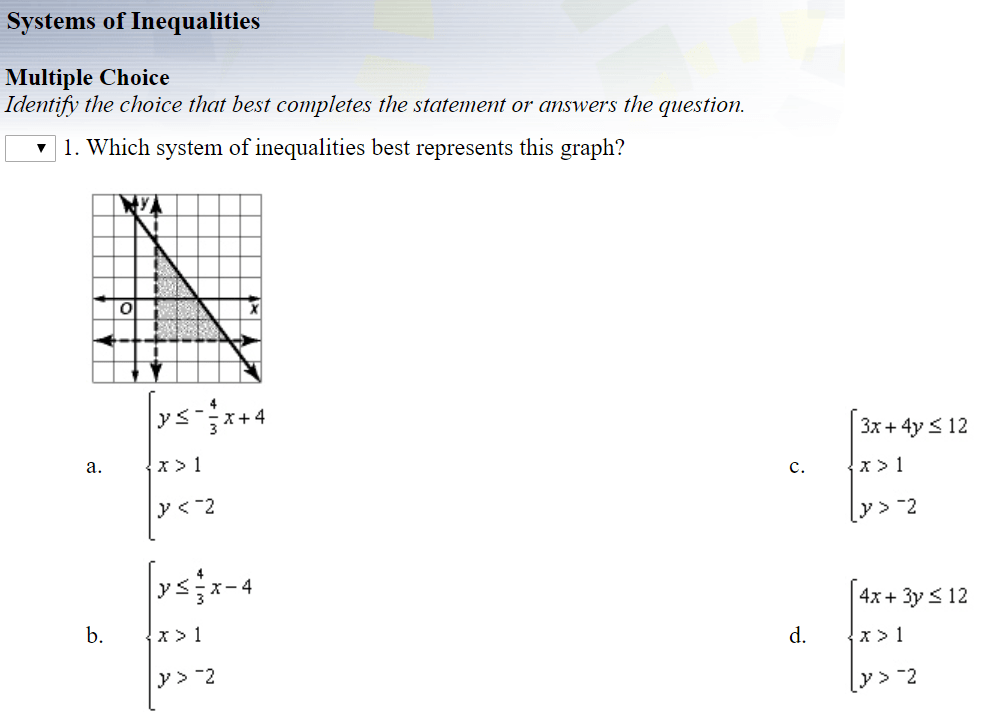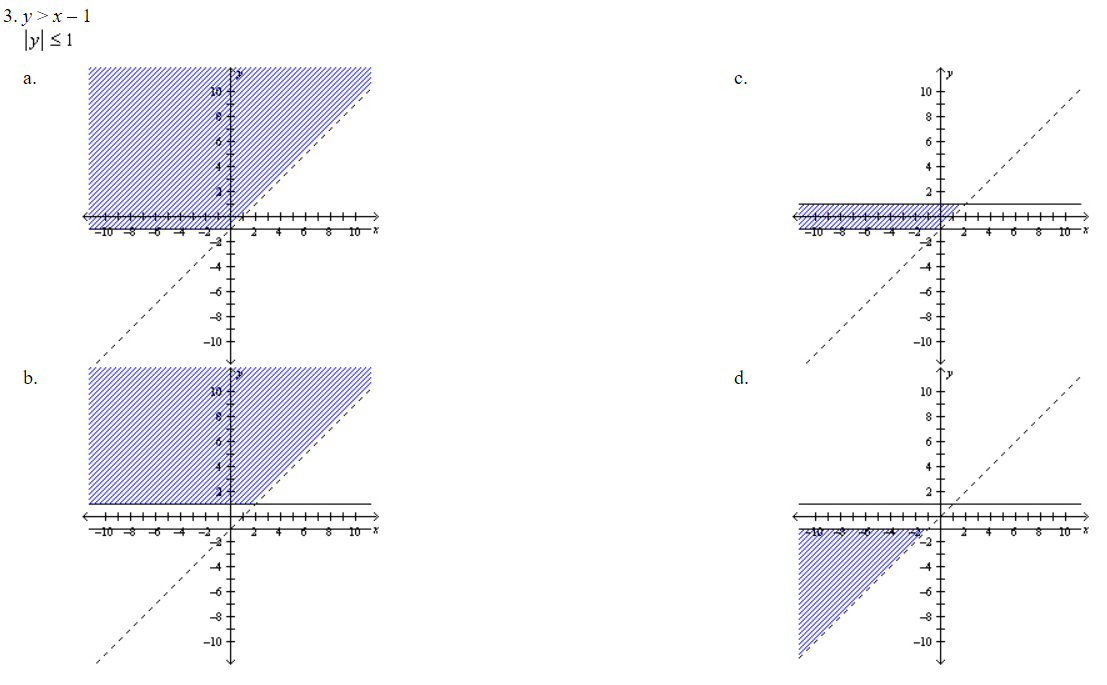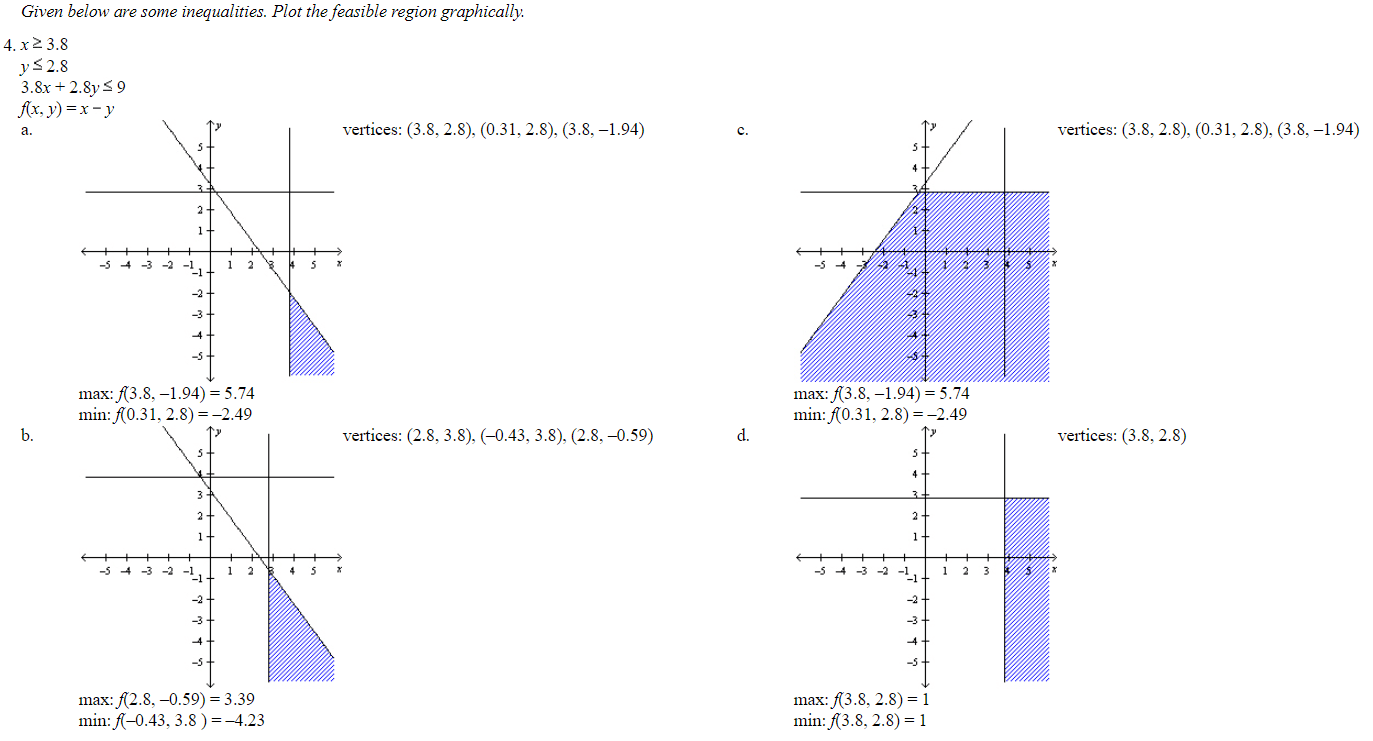# Systems of InequalitiesAnswers (1. D  2. A Both the inequalities should be plotted and the region common to both should be shaded.  3. C Plot the first inequality. Then, plot the positive and negative values of y and shade the common region.  4. A  Plot all the four graphs and shade the feasible region. Then find the coordinates of the feasible region, maximum value, and minimum value.)

(source)